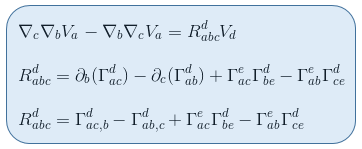## The Reimann curvature tensor

I have harpooned the great white whale of mathematics (for me at least) the Reimann curvature tensor.  Even better, I understand what curvature is, and how the Reimann curvature tensor expresses it.  Below you’ll see the nightmare of notation by which it is expressed.

Start with curvature.  We all know that a sphere (e.g. the earth) is curved.  But that’s when you look at it from space.  Gauss showed that you could prove a surface was curved just be performing measurements entirely within the surface itself, not looking at it from the outside (theorem egregium).

Start with the earth, assuming that it is a perfect sphere (it isn’t because its rotation fattens its middle).  We’ve got longitude running from pole to pole and the equator around the middle.  Perfect sphere means that all points are the same distance from the center — e.g. the radius.  Call the radius 1.

Now think of a line from the north pole to the plane formed by the equator (radius 1).  Take the midpoint of that line and inscribe a circle on the sphere, parallel to the plane of the equator.  Its radius is the half the square root of 3 (or 1.73). This comes from the right angle triangle just built with hypotenuse is 1 and  one side 1/2.   The circumference of the equator is 2*pi (remember the sphere’s radius is 1).  The circumference of the newly inscribed circle is 1.73 * pi.

Now pick a point on the smaller circle and follow a longitude down to the equator.  Call this point down1.  Move in one direction by 1/4 of the circumference of the sphere (pi/2).  Call that point on the equator down then across

Now go back to the smaller circle at the first point you picked and move in the same direction as you did on the equator by absolute distance pi/2 (not by pi/2 radians).  Then follow the longitude down to the equator.  Call that point across then down.  The two will not be the same.  Across then down is farther from down 1 than down then across.

The difference occurs because the surface of the sphere is curved, and the difference in endpoints of the two paths is exactly what the Reimann curvature tensor measures.

Here is the way the Riemann curvature tensor is notated.  Hideous isn’t it?If you’re going to have any hope of understanding general relativity (not special relativity) you need to understand curvature.

I used paths in the example, Riemann uses the slope of the paths (e.g derivatives) which makes things much more complicated.  Which is where triangles (dels), and the capital gammas (Γ) come in.

To really understand the actual notation, you need to understand what a covariant derivative actually is, which is much more complicated, but knowing what you know now, you’ll see where you are going when enmeshed in thickets of notation.

What the Riemann curvature tensor is actually saying is that the order of taking covariant derivatives (which is the same thing as the order of taking paths)  is NOT commutative.

The simplest functions we grow up with are commutative.  2 + 3 is the same as 3 + 2, and 5*3 = 3*5.  The order of the terms doesn’t matter.

Although we weren’t taught to think of it that way, subtraction is not.  5 – 3 is not the same as 3 – 5.  There is all sorts of nonCommutativity in math.  The Lie bracket is one such, the Poisson bracket  another, and most groups are nonCommutative.  But that’s enough.  I wish I’d known this when I started studying general relativity.

•BenL  On February 15, 2020 at 5:22 am

I can’t see the notation on any browser I tried (neither can I see it in the earlier test post).

•luysii  On February 15, 2020 at 9:45 am

It shows up on my machine using Apple’s Safari 13.0.5 — sorry about that

•Mark Thorson  On February 15, 2020 at 4:28 pm

I run Safari, but I couldn’t see them either. I looked at the page source, copied the link, and that wouldn’t work either. Then, I deleted the s in https and the link worked. They probably have a botched https implementation that requires supporting a security protocol you don’t support.•Ben L  On February 16, 2020 at 1:55 pm

No worries! Just found your blog and really enjoying it.

And thanks Mark!

•luysii  On February 16, 2020 at 2:51 pm

Many thanks Mark. Presently plowing through the Fermi coordinates of a body in free fall which has the result of making many of the terms in the Riemann curvature tensor zero (which is likely why they were invented by Fermi). All of them are sums of derivatives of the metric. I’m amazed that the originators could plow through this stuff without a computer algebra system.